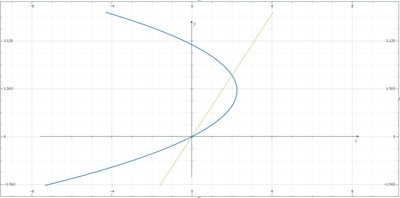# Find the area bounded by the graphs of f (y) = 3y - y^2 and g (y) = y.

## Question:

Find the area bounded by the graphs of {eq}f (y) = 3y - y^2 {/eq} and {eq}g (y) = y {/eq}.

## Area of the Region:

The region are bounded by two functions are solving its area can be done using the formula {eq}\displaystyle A=\int_{a}^{b}\left [ f(y)-g(y) \right ]dy {/eq}. Note that the limits of integration are the intersections of the two functions.

Below is the graph,The intersections of the two functions are,

{eq}3y-y^{2}=y {/eq}

{eq}y^{2}=2y {/eq}

{eq}y=0,2 {/eq}

Thus, the...

Become a Study.com member to unlock this answer! Create your account